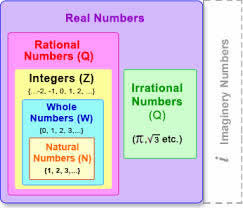# Difference between Irrational and Real numbers

##### Key Difference: An irrational number cannot be expressed in the form of a fraction with a non-zero denominator. It is just opposite of a rational number. A real number is a number that can take any value on the number line. They can be any of the rational and irrational numbers.In simple words, irrational numbers are those real numbers which cannot be expressed in the form of a fraction. Irrational numbers are just opposites of Rational numbers. In other words, Irrational numbers can be expressed as the quotient of two integers. It is important to mention that many square roots, cube roots, etc. fall in the category of irrational numbers. However, all roots are not irrational numbers. Irrational numbers can be expressed as non-terminating, non-repeating decimals. One important point to be noted is that the decimal expansion of an irrational number doesn't come to an end or repeat itself (in equal length blocks). However, it may appear in a pattern like 0.101001000100...

Real numbers define a set of values which lie between positive and negative infinity. They are used to represent various types of continuous physical quantities like distance, time and temperature. Real numbers consist of all the rational as well as irrational numbers. The system of real numbers can be further divided into many subsets:

Natural Numbers (1, 2, 3,….)

Whole Numbers (0, 1, 2, 3, 4, 5,…)

Integers (….., -3, -2, -1, 0, 1, 2, 3,…..)

Real numbers represent a quantity along a continuous line known as the Number Line. The numbers stand in one-to-one correspondence with the continuous points on the number line. They consist of whole (0, 1 ,3 ,9, 26), rational (6/9, 78.98) and irrational numbers (square root of 3, pi). Infinity does not fall in the category of real numbers. Square root of -1 is also not a real number, and therefore it is referred to as an imaginary number. A real number can be represented as a possibly infinitely long and non repeating decimal.

Comparison between Irrational and Real Number:

 Real Number Irrational Number Definition A real number is a number that can take any value on the number line. They can be any of the rational and irrational numbers. An irrational number cannot be expressed in the form of a fraction with a non-zero denominator. Number line Can be plotted on the number line. Can be plotted on the number line on the basis of proximity. Includes This includes (but is not limited to) positives and negatives, integers and rational numbers, square roots, cube roots , π (pi), etc. Square root of five, as it cannot be simplified further. 4/0, as the denominator is not non zero Pi (3.14159265358….) is the most well known irrational number. However, in certain calculations the approximate value of pi is considered Euler’s number (2.7182818284……) Golden Ratio (1.6180339887………) Important points to remember The union of the sets of rational numbers and irrational numbers. Real numbers include zero. Decimals never repeat. Decimals never end.

Image Courtesy: .formyschoolstuff.com